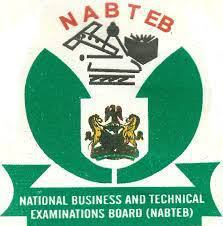## NABTEB 2019 MATHEMATICS OBJ AND ESSAY VERIFIED ANSWER_MATHS OBJ_
1ACCBABCCCA
11BCDCCAABCB
21DAAACCBDCA
===========================

(1a)
2^2x * 8^x-2 * 4^3(1-x) = 1/64

2^2x * 2^3(x-2) * 2^6(1-x) = 1/2^6
2^2x * 2^3x-6 * 2^6-6x = 2^-6
2^2x+3x-6+6-6x = 2^-6
2x +3x- 6+6-6x = -6
5x-6x = -6
-x = -6
x =6

(1b)
Log 125/81 + 2log 1^2/3
Log125/81 + 2Log5/3
Log 125/81 + Log(5/3)^2
Log 125/81 + Log 25/9
= Log ( 125/81 × 25/9 )
= Log ( 5^3 × 5^2/3^4 × 3^2 )
= Log 5^5/3^6
===========================

(2a)
A = {1,3,5}

(i) B = { x: x^2 – 7x + 12}
= { x: (x^2 – 3x) – (4x + 12)}
= { x: x(x-3) – 4(x-3)}
= { x: (x-4) (x-3) = 0}
= { x: x-4=0 or x-3=0}
B = {3,4}
(ii) AnB = {1,3,5}n{3,4}
= {3}

(2bi)
Sum of interior angles = (2n-4)90
(2n-4)90 = 1080
2n-4 = 1080/90
2n-4 = 12
2n = 12+4
2n = 16
n = 16/2 = 8
(2bii)Interior angles = 1080/80 =135degree
Exterior + Interior = 180
Exterior + 135 = 180
Exterior = 180-135 = 45degree
===========================

(3a)
120x + 10(base 4) = 110(base 4)

(1*x^2) + (2*x^1) + (1*x^0) + (1*4^1) + (0*4^1)= (1*4^0) + (1*4^1) + (0*4^0)

x^2 + 2x + 5 = 20
x^2 +2x + 5 -20 =0
x^2 + 2x -15 = 0
(x^2 + 5x)-(3x-15) = 0
x(x+5)-3(x+5) =0
(x-3)(x+5) =0
x -3=0 or x+5=0
x =3 or x= -5
Therefore , x=3

(3b)
Length of chord = 2rsinΦ/2
= 2×6sin80/2
= 12sin40
= 12 × 0.6428
L = 7.7cm
===========================

(4a)
Proportion = 1^1/2 : 2 : 3
= 3/2 : 2 : 3
Sum = 3/2 + 2 + 3 = 3/2 + 5/1
= 3+10/2 – 13/2

Let the total length be L
Longest piece = 20.7cm
= 3/13/2 × L = 20.7
= 3×2/13 × L = 20.7
6L = 13×20.7
6L = 269.1
L = 269.1/6
L = 44.85cm

Hence, the shortest piece
L = 3/2/13/2 × 44.85
= (3/2 × 2/13) × 44.85
= 3/13 × 44.85
= 10.35cm

(4b)
PRT= ΔPQR { Alternative segment is equal }
ΔPQR =58°
From ΔPQR
ΔPQR + ΔQRP + ΔPRQ =180°
58+65+ΔPRQ = 180°
PRQ =180-123 =57°
ΔPRQ = 57°
===========================

(5a)
Chika = x+10

CHECK OUT:  NABTEB 2019 ENGLISH LANGUAGE OBJ AND ESSAY VERIFIED ANSWER

In five years time
Chika = (x+10)+5 = x+15
x+15 = 2(x+5)
x+15 = 2x+10
2x-x = 15-10
x = 5years

Chika = x+10
= 5+10 =15years
Chika = 15years

(5b)
21m^2 + 5m =6
21m^2 + 5m -6=0
(21m^2 + 14m)-(9m-6)=0
7m(3m+2)-3(3m+2)=0
(7m-3)(3m+2)=0
7m-3=0 or 3m+2=0
7m=3 or 3m= -2
m = 3/7 or m = -2/3
===========================

(6a)
PQ = 420km

Latitude of P =30°N
Latitude of Q =?

PQ = Φ/360 × 5πR
420=Φ/360 ×2×22/7×6400
420=281600Φ/2520
281600Φ=420×2520
281600Φ= 1058400
Φ=1058400/281600
Φ=3.76
Φ=4°{ nearest degree }

Q°N – 30 =4
Q = 4+30
Q=34°N

(6b)
(1- √3)/√5 – √2) + (1+ √3/√5 + √2)

= (1 – √3)(√5 + √2) + (1+√3)(√5 – √2)/ (√5 – √2)(√5 + √2)

= (√5 + √2 – √15 – √6 + √5 – √2 + √15 – √6)/ ( 5 + √10 – √10 – 2)

= 2√5 – 2√6/5-2 = 2(√5 – √6)/3
===========================

(7a)
Given that;
A = 3.48, B = 21.75 and C = 0.265
√BC/A² = √21.75*0.265/(3.48)²

Tabulate

Under; No
21.75

0.265

(21.75*0/265)½

(3.48)²

0.1983

Under; Log
1. 3375
+
T. 4232/0.7607
=0.7607÷2
=0.3804. Numerator

0.5416*2

0.3804. Denumerator

0.3804
1.0832/T. 2972

√21.75*0.265/(3.48)²

(7b)
s+ 2 = 2 +t/2-t
(s+2)(2-t)= 2+t
2s – st + 4 -2t=2+t
2s+4-2=t+st+2t
2s+2=3t+st
2s+2=t(3+s)
t =2s+2/3+s
t =2(s+1)/s+3

Given s=10.5
t =2(10.5+1)/10.5+3
= 2×11.5/13.5 =1.7
t=1.7
===========================

(8a)
Interior angle = 140
Exterior angle = 180-140=40

Number of sides =360/40=9

Therefore,
2p+1=9
2p=9-1
2p=8
p =8/2=4
p =4

(8bi)
U=90
n(FnT)=5
n(TnA)=8
n(FnA)=15
n(F)=30
n(T)=25
n(FnTnA)=4
n(FnTnA)^c=x

Football (F) only = 30-(1+4+11)
=30-16=14
Tennis (T) only = 25-(1+4+4)
= 25-9=16
Athletic (A) only = 18

(8bii)
14+16+18+11+4+1+4+x=90
= 68+x=90
x=90-68
x=22
===========================

(9a)
H = height of the tree

From ΔTCA
tan45 = H/x+3
H=(x+3)tan45……(i)

From ΔTCB
tan54 = H/x
H=xtan54……(ii)

Equating (i) and (ii)
(x+3)tan45 – xtan54
x+3= 1.3764x
1.3764x – x=3
0.3764x=3
x=3/0.3764
x=7.97m

CHECK OUT:  NABTEB 2019 BIOLOGY PRACTICAL VERIFIED ANSWER

Hence ,
Height of the tree
H=xtan54
= 7.97tan54
= 7.97 × tan54
= 7.97 × 1.3764
H=10.97m
===========================

(11a&11b)
===========================

(12a)
Tabulate

Age(x) (in months) – 202,168,158,151,135,116

Frequency (f) – 1,1,1,1,1,1

Fx – 202,168,158,151,135,116

Ef = 6

Efx = 930months

(i) Mean = Efx/Ef = 930/6 =155months
= 12years 11months
(ii) Median = 158+151/2 = 309/2=154.5
= 154months 15days

(12b)
Price tag of the car = N2,400,000.00
Selling price of the old car = N350,000.00
Balance of the new car = N2,400,000 – N350,000
= N2,050,000
10% of 2,050,000 = 10/100 × 2,050,000
= N205,000
Total balance of the new car price = N2,050,000 – N205,000
= N2,225,000

Monthly installment payment for 54months = N2,225,000/54
= N41,759.26
===========================

(13a)
P=N500,000.00 , R=5% , T=4years
That’s = PRT/100 = 500,000 × 5 × 4/100 = 5,000 × 20
= N100,000
Amount at the end of 4yrs
A= P+I = 500,000 + 100,000 N600,000

(13b)
Total cost of the motor tyre = 15,000 × 100 = 1,500,000.00
Selling price of 45 = N18,000 × 45 = N810,000.00
Selling price of 40 = N20,000 × 40 = N800,000.00
Selling price of the 85 tyres = N810,000 + N800,000
= N1,610,000
Profit = N1,610,000 – 1,500,000 + 285,000
= N110,000 + N285,000 = N395,000
% Profit = 395,000/1,500,000 × 100
= 26.33%Join Our Telegram Channel |Whatsapp Our Admins: Click Any Name Mr.Paul or Mr.Henry

### Comment

Posted by on 27th May 2019.

Categories: NABTEB